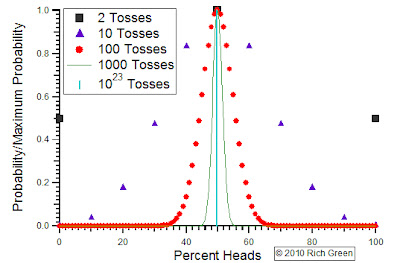Friday, November 12, 2010

Fluctuations

This post is part of a series,Nonsense and the Second Law of Thermodynamics The previous post is entitled Entropy and Statistical Dynamics.

The second law of thermodynamics works because of the statistics of very large numbers. Consider a bouncing ball: as it bounces, it dissipates heat and eventually does not bounce as high.Eventually the ball is stationary, but the room is imperceptibly hotter. Energy has been conserved.

The reverse process would also conserve energy. All of the molecules in the air could simultaneously come together and impart momentum to the ball causing it to bounce higher and higher. The energy would come from the heat in the room, and the ball would bounce higher.

The statistics are weighted against such an event ever happening.  Consider also that the statistics in question involve extremely large numbers.

Thermodynamic systems do fluctuate from their most probable distribution, but the fluctuations are in general negligible.  Fluctuations are on the order of the square root of N, but remember N is very large: the square root of 1023 is 3 x 1011, which is 3 x 10 -10 percent of N, a negligible probability.

To illustrate: imagine the simple experiment of tossing a coin to see how many heads one gets.  If one tosses the coin one time, there is an equal chance of getting heads or tails. If one tosses the coin two times, the most probable event is that one gets heads 50% of the time.  Consider the possibilities:

Tails, Tails

There are four equally probable outcomes.  For two of the outcomes, one gets heads 50% of the time. There is a 50% probability of getting this outcome, which is the most probable outcome. The most probable event is that heads comes up half of the time.  In the case of four tosses, it is equally likely not to get the most probable result.

There is a 25% chance one gets two heads, and a 25% chance one gets two heads, or a 50% chance that one does not get 50% heads.

One can calculate these probabilities for tossing the coin more times through the binomial distribution:

Probability of getting K heads in N tosses = N!/K! x (N-K)!

The "!" indicates factorial.  N! means N x (N-1) x (N-2) ... x 1. For example:

5! = 5 x 4 x 3 x 2 x 1 = 120

The plot shows the binomial distribution for N=2,10,100, and 1000.  The probabilities have been normalized by the maximum probability to show them on the same scale.

Notice that as N gets larger, the distribution gets tighter.  The probability of getting a result far from 50% drastically reduces as N increases.

Most of the distribution is contained within the square root of N of the most probable result.  If N=10, the square root of 10 is about 3.2, or about 30% of 10.  Most of the distribution falls between 20% and 80% chance of getting heads.

For N=100, the square root of 100 is 10, or 10% of 100.  Most of the distribution falls between 40-60%.  As N increases, the distribution get tighter and tighter.  For N = 1023, the distribution is for all intents and purposes a delta function.  The square root of N is an insignificant percentage.

To see how all of these statistics apply to molecules, one has to understand a little bit about partition functions, the subject of the next post.

Sources
• Atkins, P. W. Physical Chemistry, W. H. Freeman and Company, New York, 3rd edition, 1986
• McQuarrie, Donal d A., Statistical Thermodynamics,  University Science Books, Mill Valley, CA, 1973
• Bromberg, J. Philip, Physical Chemistry, Allan and Bacon, Inc., Boston, 2nd Edition, 1984
• Anderson, H.C., Stanford University, Lectures on Statistical Thermodynamics, ca. 1990.
Contents

1 comment:

Fastest train in the world said...

Top 12 Fastest Trains in the World Here are the world’s fastest trains in commercial service, ranked by their operating speed Top 12 Fastest Trains in the World